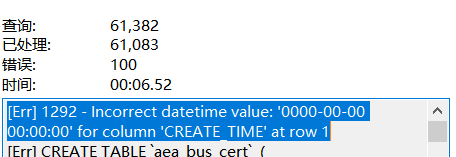Python的多任务编程

``````from multiprocessing import Process
import os,time

l=[]
stop=time.time()
def work():
global stop
time.sleep(2)
stop=time.time()

def test1():
for i in range(400):
l.append(p)
p.start()

def test2():
for i in range(400):
l.append(p)
p.start()

for p in l:
p.join()

if __name__ == '__main__':
print("CPU Core:",os.cpu_count()) #本机为4核
print("Worker: 400") #测试线程数

start=time.time()
test1()
while (active_count>1):
continue
test1_result=stop-start

start=time.time()
l=[]
test2()
while (active_count>1):
continue

print('Thread run time is %s' %(test1_result))
print('Thread join run time is %s' %(stop-start))``````

``````Thread run time is 4.829492807388306
Thread join run time is 2.053645372390747``````

``````rom multiprocessing import Process
import os,time

l=[]
def work():
res=0
for i in range(100000000):
res*=i

def test1():
for i in range(4):
p=Process(target=work)
l.append(p)
p.start()

def test2():
for i in range(4):
l.append(p)
p.start()

for p in l:
p.join()

if __name__ == '__main__':
print("CPU Core:",os.cpu_count()) #本机为4核
print("Worker: 4") #工作线程或子进程数

start=time.time()
test1()
while (l[len(l)-1].is_alive()):
continue
stop=time.time()
print('Process run time is %s' %(stop-start))

start=time.time()
l=[]
test2()
while (l[len(l)-1].is_alive()):
continue
stop=time.time()
print('Thread run time is %s' %(stop-start))
``````

``````CPU Core: 4
Worker: 4
Process run time is 11.030176877975464

`````` import asyncio
import time

async def work(i):
await asyncio.sleep(2)
print('===>',i)

async def main():
start=time.time()
l=[]
for i in range(400):
l.append(p)

for p in l:
await p

stop=time.time()
print('run time is %s' %(stop-start))

asyncio.run(main())``````

``run time is 2.0228068828582764``

``````from multiprocessing import Process
import asyncio
import os,time

l=[]
async_result=0
async def work1():
res=0
for i in range(100000000):
res*=i

# 协程入口
async def async_test():
m=[]
for i in range(4):
m.append(p)

for p in m:
await p

async def async_test1():

def async_run():
asyncio.run(async_test1())

# 多进程入口
def test1():
for i in range(4):
p=Process(target=async_run)
l.append(p)
p.start()

if __name__ == '__main__':
print("CPU Core:",os.cpu_count()) #本机为4核
print("Worker: 4") #工作线程或子进程数
start=time.time()
asyncio.run(async_test())
stop=time.time()

print('Asyncio run time is %s' %(stop-start))

start=time.time()
test1()
while (l[len(l)-1].is_alive()):
continue
stop=time.time()

print('Process Asyncio run time is %s' %(stop-start))``````

``````CPU Core: 4
Worker: 4
Asyncio run time is 18.89663052558899
Process Asyncio run time is 10.865562438964844``````

``````from multiprocessing import Process
import os,time

l=[]
# 仅计算
def work1():
res=0
for i in range(100000000):
res*=i

# 仅输出
def work2():
time.sleep(2)
print('===>')

# 多进程，仅计算
def test1():
for i in range(4):
p=Process(target=work1)
l.append(p)
p.start()

# 多进程，仅输出
def test_1():
for i in range(400):
p=Process(target=work2)
l.append(p)
p.start()

# 多线程，仅计算
def test2():
for i in range(4):
l.append(p)
p.start()

for p in l:
p.join()

# 多线程，仅输出
def test_2():
for i in range(400):
l.append(p)
p.start()

for p in l:
p.join()

if __name__ == '__main__':
print("CPU Core:",os.cpu_count()) #本机为4核

start=time.time()
test1()
while (l[len(l)-1].is_alive()):
continue
stop=time.time()
test_result=stop-start

start=time.time()
l=[]
test_1()
while (l[len(l)-1].is_alive()):
continue
stop=time.time()
test1_result=stop-start

start=time.time()
l=[]
test2()
while (l[len(l)-1].is_alive()):
continue
stop=time.time()
test2_result=stop-start
start=time.time()
l=[]
test_2()
while (l[len(l)-1].is_alive()):
continue
stop=time.time()
test3_result=stop-start
print('Process run time is %s' %(test_result))
print('Process I/O run time is %s' %(test1_result))
print('Thread run time is %s' %(test2_result))
print('Thread I/O run time is %s' %(stop-start))
``````

``````Process run time is 10.77662968635559
Process I/O run time is 2.9869778156280518
Thread I/O run time is 2.024587869644165
``````blmius
1年前
MySQL:[Err] 1292 - Incorrect datetime value: ‘0000-00-00 00:00:00‘ for column ‘CREATE_TIME‘ at row 1Jacquelyn38
2年前
2020年前端实用代码段，为你的工作保驾护航helloworld_34035044
11个月前

​在我们用皕杰报表工具设计填报报表时，如何在新增行里自动增加id呢？能新增整数排序id吗？目前可以在新增行里自动增加id，但只能用uuid函数增加UUID编码，不能新增整数排序id。uuid函数说明：获取一个UUID，可以在填报表中用来创建数据ID语法：uuid()或uuid(sep)参数说明：sep布尔值，生成的uuid中是否包含分隔符''，缺省为Stella981
1年前
Python3：sqlalchemy对mysql数据库操作，非sql语句
Python3：sqlalchemy对mysql数据库操作，非sql语句python3authorlizmdatetime2018020110:00:00coding:utf8'''Stella981
1年前
KVM调整cpu和内存Stella981
1年前
Python之time模块的时间戳、时间字符串格式化与转换
Python处理时间和时间戳的内置模块就有time，和datetime两个，本文先说time模块。关于时间戳的几个概念时间戳，根据1970年1月1日00:00:00开始按秒计算的偏移量。时间元组（struct_time），包含9个元素。 time.struct_time(tm_yWesley13
1年前
mysql设置时区
mysql设置时区mysql\_query("SETtime\_zone'8:00'")ordie('时区设置失败，请联系管理员！');中国在东8区所以加8方法二：selectcount(user\_id)asdevice,CONVERT\_TZ(FROM\_UNIXTIME(reg\_time),'08:00','0Wesley13
1年前
00：Java简单了解Stella981
1年前Wesley13
1年前
MySQL部分从库上面因为大量的临时表tmp_table造成慢查询Lv1

16

2

9